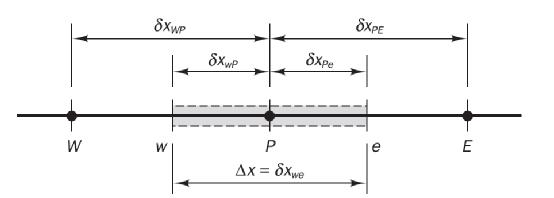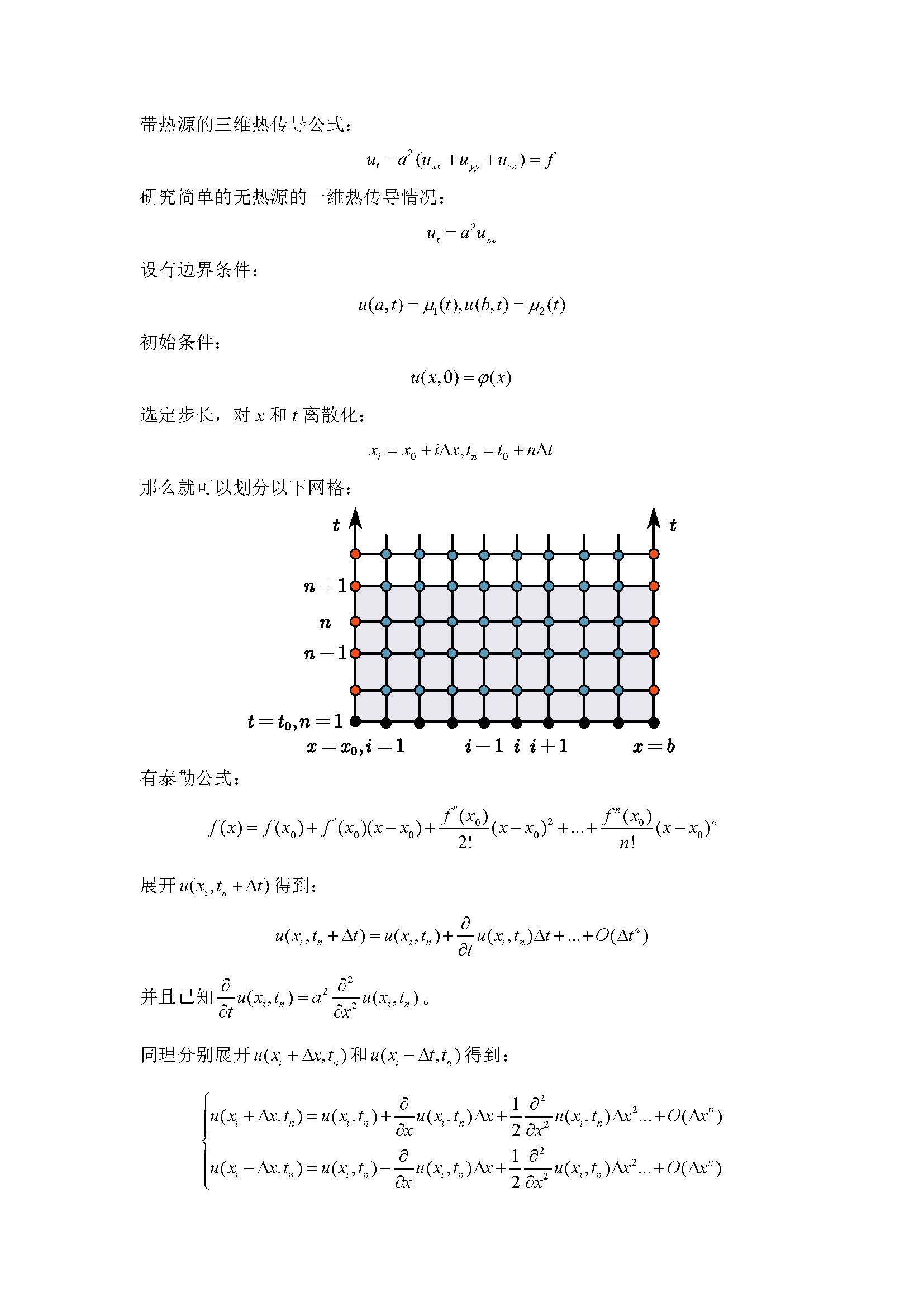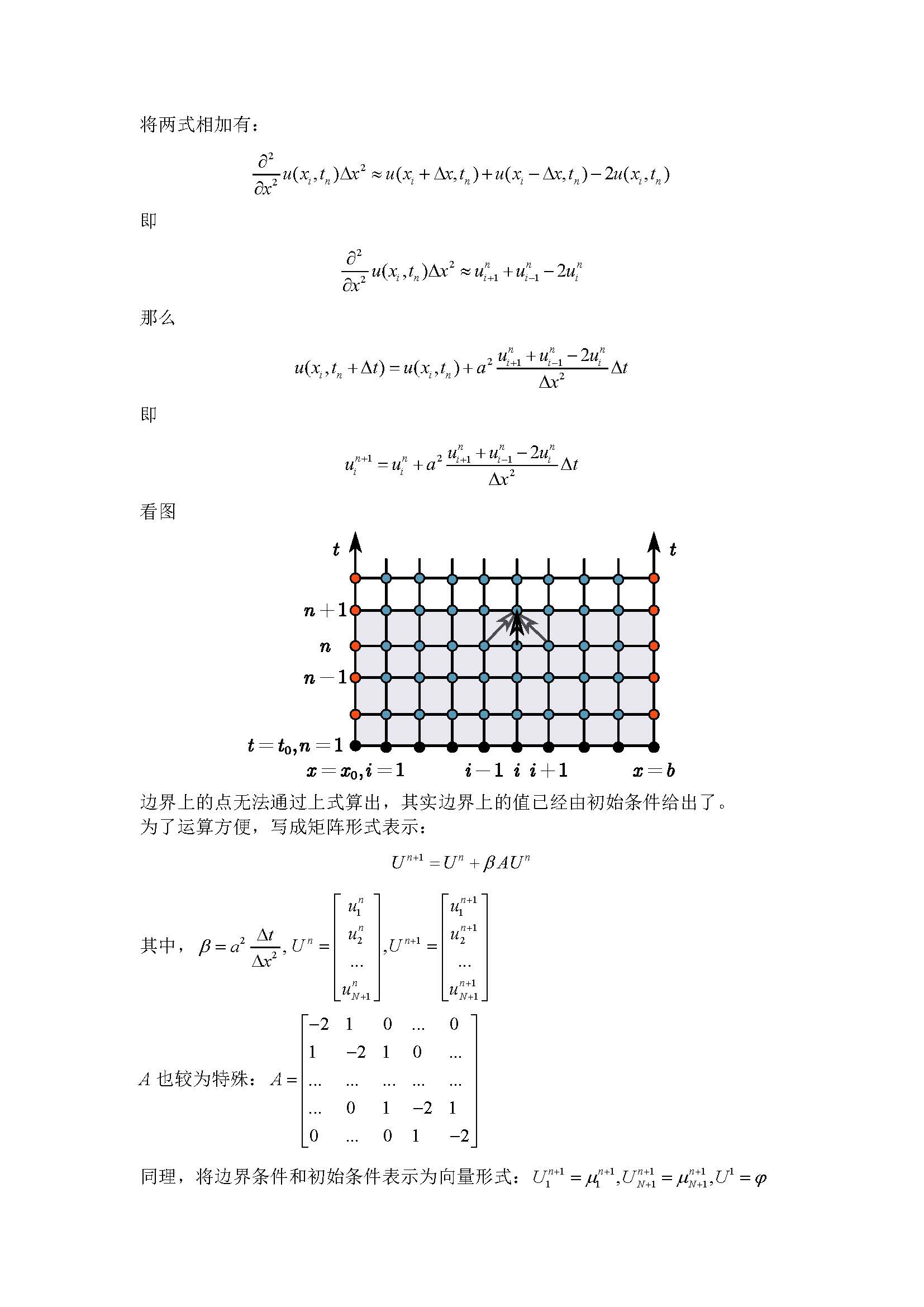• 求解一维热传导问题/给定边界条件/纯手打代码/参考书籍为数值计算方法matlab
• 1一维热传导问题与C++描述1.1理论推导扩散问题的通用方程可以写为： ∇∇Γ+S=0(1)\begin{equation} \nabla\nabla\Gamma +S = 0\tag{1} \end{equation} 一维热传导问题可以讲上式子简化为来： ddx(Γdϕdx)+S=0...
1一维热传导问题与C++描述
1.1理论推导
扩散问题的通用方程可以写为：

∇∇Γ+S=0(1)

\begin{equation}
\nabla\nabla\Gamma +S = 0\tag{1}
\end{equation}

一维热传导问题可以讲上式子简化为来：

ddx(Γdϕdx)+S=0(2)

\begin{equation}
\frac{d}{dx}(\Gamma\frac{d\phi}{dx}) +S = 0\tag{2}
\end{equation}
将其在网格上离散可以得到下图的控制体单元表示：将1.2式在网格上积分并利用Gauss定理就可以得到：

\begin{equation}
\int_{{\Delta}V}\frac{d}{dx}(\Gamma\frac{d\phi}{dx})dV+\int_{{\Delta}V}SdV
=({\Gamma}A\frac{d\phi}{dx})_{e}-({\Gamma}A\frac{d\phi}{dx})_w+\bar S{\Delta}V=0\tag{3}
\end{equation}

Γw
${\Gamma}_{w}$与

Γe
${\Gamma}_{e}$可以用线性插值的方法得到。1.3再在w,e面上的值即可以写为

\begin{equation}
({\Gamma}A\frac{d\phi}{dx})_{e}={\Gamma}_{e}A_e\frac{\phi_E-\phi_P}{{\delta}_{EP}}\tag{4}
\end{equation}

\begin{equation}
({\Gamma}A\frac{d\phi}{dx})_{w}={\Gamma}_{w}A_w\frac{\phi_P-\phi_W}{{\delta}_{PW}}\tag{5}
\end{equation}
源项可以用

S¯ΔV=Su+Spϕp
$\bar S{\Delta}V=S_{u}+S_{p}\phi_{p}$代替，则式3可以写为：

ΓeAeϕE−ϕPδEP−ΓwAwϕP−ϕWδPW+Su+Spϕp=0(6)

\begin{equation}
{\Gamma}_{e}A_e\frac{\phi_E-\phi_P}{{\delta}_{EP}}-{\Gamma}_{w}A_w\frac{\phi_P-\phi_W}{{\delta}_{PW}}+S_{u}+S_{p}\phi_{p}=0\tag{6}
\end{equation}
整理就得到：

aPϕP=aWϕW+aEϕE+Su(7)

\begin{equation}
a_{P}\phi_{P}=a_{W}\phi_{W}+a_{E}\phi_{E}+S_u\tag{7}
\end{equation}

其中:

aW
$a_{W}$

aE
$a_{E}$

aP
$a_{P}$

ΓWδxWPAW
$\frac{\Gamma_{W}}{{\delta}{x}_{WP}}A_{W}$

ΓEδxPEAE
$\frac{\Gamma_{E}}{{\delta}{x}_{PE}}A_{E}$

aW+aE+Su
$a_{W}+a_{E}+S_u$
1.2一维传热的小case其中

δx
$\delta x$为

0.1m
$0.1m$，

k=1000w/m⋅s
$k=1000w/m\cdot s$，截面积

A=0.01m2
$A = 0.01m^{2}$。  根据公式7我们可以直接得到2、3、4节点处的方程组的系数。而对于边界1点处，可以类比公式6:

ΓAT2−T1δ21−ΓATP−TAδ1A=0(8)

\begin{equation}
{\Gamma}A\frac{T_2-T_1}{{\delta}_{21}}-{\Gamma}A\frac{T_P-T_A}{{\delta}_{1A}}=0\tag{8}
\end{equation}

同理对于5点我们也有:

ΓATB−T5δ5B−ΓAT5−T4δ54=0(8)

\begin{equation}
{\Gamma}A\frac{T_B-T_5}{{\delta}_{5B}}-{\Gamma}A\frac{T_5-T_4}{{\delta}_{54}}=0\tag{8}
\end{equation}

对于这个case我们所需的全部都已经得到，可以用C++编程语言进行具体的求解。
1.3 C++描述
1.3.1一个有用的数据结构
观察公式7、8、9我们发现实际上对于结构化网格，实际上网格任意一点所需求解的值都仅与相邻网格或者边界上的值相关，我们也就可以用

aPϕP=aWϕW+aEϕE+aNϕN+aSϕS+aTϕT+aBϕB+Su(7)

\begin{equation}
a_{P}\phi_{P}=a_{W}\phi_{W}+a_{E}\phi_{E}+a_{N}\phi_{N}+a_{S}\phi_{S}+a_{T}\phi_{T}+a_{B}\phi_{B}
+S_u\tag{7}
\end{equation}

来表示任意一个网格节点处的离散化的控制方程。在这里就可以定义一个非常哟用的类或者结构体来储存这些系数。
#ifndef _LinearCofs_
#define _LinearCofs_

using namespace std;

class LinearCofs
{
public:
double ap_, ae_, aw_, an_, as_, at_, ab_;
double b_;

LinearCofs()
:ap_(0), ae_(0), aw_(0), an_(0), as_(0), at_(0), ab_(0), b_(0)
{}
{

}

LinearCofs(double ap, double ae, double aw, double an, double as, double at, double ab, double b)
:ap_(ap), ae_(ae), aw_(aw), an_(an), as_(as), at_(at), ab_(ab), b_(b)
{}

};

#endif
即使在后面的三维问题的求解中我们也可以利用上述类。
1.3.2 求解过程

#include <iostream>
#include <vector>

#include "LinearCofs.h"

#include "Matrix.h"

const double  N = 5;
const double k_ = 0.5;
const double A_ = 1;
const double L_ = 0.02;
const double TA_ = 100;
const double TB_ = 500;

double deltx = L_ / N;

using namespace std;

void InerField(vector<LinearCofs>&  lcDiffusion1D)
{

for( int i = 1; i < N - 1; i++)
{
lcDiffusion1D[i].ae_ = -k_ / deltx * A_;
lcDiffusion1D[i].aw_ = -k_ / deltx * A_;
lcDiffusion1D[i].ap_ = -(lcDiffusion1D[i].ae_ + lcDiffusion1D[i].aw_);
}

}

void BoundaryCondition(vector<LinearCofs>&  lcDiffusion1D)
{
double deltx = L_ / N;

lcDiffusion1D.ae_ = -k_ / deltx * A_;
lcDiffusion1D.aw_ = 0.0;
lcDiffusion1D.ap_ = -(lcDiffusion1D.ae_ + lcDiffusion1D.aw_)+2 * k_ / deltx * A_;

lcDiffusion1D[N - 1].ae_ = 0.0;
lcDiffusion1D[N - 1].aw_ = -k_ / deltx * A_;
lcDiffusion1D[N - 1].ap_ = -(lcDiffusion1D[N - 1].ae_ + lcDiffusion1D[N - 1].aw_)+2 * k_ / deltx * A_;
}

void CofsToMatrix(Matrix&   mDiffusion1D, vector<LinearCofs>&   lcDiffusion1D)
{

for(int i = 0; i < N; i++)
{
for(int j = 0; j < N; j++)
{
mDiffusion1D(i,j) = 0.0;
}
}

mDiffusion1D(0,0) = lcDiffusion1D.ap_;
mDiffusion1D(0,1) = lcDiffusion1D.ae_;

for (int i = 1; i < N-1 ; i++)
{

mDiffusion1D(i,i-1) = lcDiffusion1D[i].aw_;
mDiffusion1D(i,i) = lcDiffusion1D[i].ap_;
mDiffusion1D(i,i+1) = lcDiffusion1D[i].ae_;
}

mDiffusion1D(N-1,N-2) = lcDiffusion1D[N-1].aw_;
mDiffusion1D(N-1,N-1) = lcDiffusion1D[N-1].ap_;

}

void CofsToB_(vector<LinearCofs>&   lcDiffusion1D)
{
lcDiffusion1D.b_ = 2 * k_ / deltx*A_ * TA_;
lcDiffusion1D[N-1].b_ = 2 * k_/ deltx*A_ * TB_;

}

int main()
{

Matrix  mDiffusion1D(N,N);

vector<LinearCofs>  lcDiffusion1D;
AllocateDim(lcDiffusion1D, N);

InerField(lcDiffusion1D);

BoundaryCondition( lcDiffusion1D );

CofsToMatrix(mDiffusion1D, lcDiffusion1D);

CofsToB_( lcDiffusion1D );

cout << "Matrix A is: " << endl;

cout << mDiffusion1D;

vector<double> x(N),b(N);
for(int i = 0; i < N; i++)
{
b[i] = lcDiffusion1D[i].b_;
}

mDiffusion1D.GaussElimination(x, b);

cout << endl;
cout << " The solution is " << endl;
for(int i = 0; i < N; i++)
{

cout <<"x["<<i<<"]="<< x[i] <<" ";

}

cout << endl;

system("pause");

return 0;
}

这里第一个函数InerField()是对内部的3\4\5节点赋值，BoundaryCondition()函数则是对两端的1\5点赋值，CofsToMatrix()是将得到的方程组放到矩阵中求解，矩阵求解器可以再我前面的文章中得到,大家可以直接复制，然后include 到头文件中。我们的矩阵求解器有两款线性方程组求解的算法，当然后面我们对于二维问题，因为比较复杂，会有一个gauss-seidel的求解器，会在后面给出那种直接求解不需要赋值到矩阵中的方法。  利用上述算法我们可以得到每个节点处的值了。
展开全文C++
• 1.一维热传导问题：模型始端是第二类边界条件，末端是第三类边界条件，这离散方程用MATLAB应该怎样编程？ 2.一维热传导问题：模型中不同材料结构的接触面处的离散方程用MATLAB应该怎样编程？matlab
• Matlab 求解一维热传导方程 %%传热模型 clear ; g = ‘squareg’; %定义以为正方形 b = ‘squareb1’; %定义边界条件为零 c = 1; a = 0; f = 0; d = 1; %产生初始三角网格 [p,e,t]=initmesh(g); %定义初始条件 u0 = ...


Matlab 求解一维热传导方程

%%传热模型 clear ; g = ‘squareg’; %定义以为正方形 b = ‘squareb1’; %定义边界条件为零 c = 1; a = 0; f = 0; d = 1; %产生初始三角网格 [p,e,t]=initmesh(g); %定义初始条件 u0 = zeros(size(p,2),1); ix=find(sqrt(p(1,:).^2+p(2,:). ^2)<0.4); u0(ix)=1; %在时间段0~0.1 的20个点上求解 nframe = 20; tlist = linspace(0,0.1,nframe); u1 = parabolic(u0,tlist,b,p,e,t,c,c,f,d); %动画效果 for j = 1:nframe pdesurf(p,t,u1(:,j)); mv(j) = getframe; end movie(mv,10);
展开全文• MATLAB代码 clear a = 1; dx = 0.01;%dx要大 x = 0:dx:1;... % 温度随时间传导的动画 plot(x,u(:,n+1)) axis([x(1) x(end) 0 1]) getframe; end % [T,X] = meshgrid(t,x); % surf(X,T,u); % shading interpMATLAB代码
clear
a = 1;
dx = 0.01;%dx要大
x = 0:dx:1;
dt = 0.00005;%dt要小
t = 0:dt:1;
u = zeros(length(x),length(t));
% 初始条件,x的函数
u(:,1) = sin(pi*x);
% 边界条件,t的函数
m1 = 1 + 0.0*sin(t);
m2 = 1 - 0.2*sin(10*t);
% 构造A矩阵
A = -2*eye(length(x)) + diag(ones(1,length(x)-1),1) + diag(ones(1,length(x)-1),-1);
for n = 1:length(t) - 1
u(:,n+1) = u(:,n) + a^2*dt/dx^2*A*u(:,n);
u(1,n+1) = m1(n+1);
u(end,n+1) = m2(n+1);

% 温度随时间传导的动画
plot(x,u(:,n+1))
axis([x(1) x(end) 0 1])
getframe;
end
% [T,X] = meshgrid(t,x);
% surf(X,T,u);


展开全文matlab
• 一维热传导问题的数值解法及 MATLAB 模拟;一维热传导问题的数值解法及 MATLAB 模拟;学 海 无 涯 ;一维热传导问题的数值解法及 MATLAB 模拟;一维热传导问题的数值解法及 MATLAB 模拟;一维热传导问题的数值解法及 ...
• 利用差分法对数理方程的多个较复杂的一维热传导问题进行分析,并进行数值计算,给出了直观的图象。
•文档
• 学 海 无 涯 昆 明 学 院 2015 届毕业设计论文 设计论文题目 一维热传导问题的数值解法及其 MATLAB 模拟 子课题题目 无 姓 名 伍有超 学 号 201117030225 所 属 系 物理科学与技术系 专业年级 2011 级物理学 2 班 ...
• 一维热传导方程热源反问题基于最小二乘法的正则化方法研究论文
• 一维热传导模型下CRAM存储元结构及其与CMOS电路热兼容问题探索，向宏酉，王嘉赋，从硫系化合物随机存取存储器Chalcogenide RAM(CRAM)的存储原理出发，建立存储元一维多层热传导结构模型。根据一维条件下存储元的读写...
• 一维热传导方程为例，对离散正则化方法进行了阐述，并进行了数值模拟。结果表明离散正则化方法的数值模拟结果与真实结果之间的误差较小，并且.随着离散密度的增加，误差越来越小。这种方法避免引入泛函，使用起来...
• 这是个动态边界二维热传导问题。 问题出现的背景是在水浴中淬火的钢坯。 该问题具有狄利克雷边界条件； 它们随着水浴温度的变化而不断变化。 使用的配方是 FTCS。 可以通过改变“ControlPanel”文件中的属性来...matlab
• MATLAB编辑一维热传导方程的模拟程序 求解下列热传导问题 程序 function heat_conduction) %一维齐次热传导方程 options={'空间杆长L'空间点数N' '时间点数M'扩散系数alfa'稳定条件的值lambda(取值必须小于0.5,};...
• 一维热传导方程，一维热传导方程，一维热传导方程，一维热传导方程，一维热传导方程
• 一维热传导型半导体器件的有限体积元逼近及分析.pdf
• 求解下列热传导问题: 2T 1 T 门 c , 2 0 0 z L z t T乙0 1 z2 T 0,t 1, T L,t 0 L 1, 1 程序 fun ctio n heat_c on ductio n) % 一维齐次热传导方程 稳定条options={'空间杆长L'空间点数N'时间点数M'扩散系数alfa' ...
•MATLAB
• 题入手，设计了个轻量级热传导串行算法，并将此算法在GPU上的实现并行 化。在GPU中将粒子按照数目规模划分为若干个网格，每执行一步共享内存中 数据同步次。200000步后计算结果数据传回CPU，计算出每个粒子的...
• 学过计算流体力学的朋友，在控制方程数学性质方面，可能会遇到控制方程类型证明问题，例如：一维热传导方程、拉普拉斯方程、一维波动方程推导，建议多从克莱默法则入手，构造方程组，求解特征线。 ...
学过计算流体力学的朋友，在控制方程数学性质方面，可能会遇到控制方程类型证明问题，例如：一维热传导方程、拉普拉斯方程、一维波动方程推导，建议多从克莱默法则入手，构造方程组，求解特征线。二阶的导数部分，采用一阶导数替换，令,再结合全微分方程即可。
展开全文• MATLAB 编辑 一维 热传 导方程的模拟程序 求解下列热传导问题: 2T z2 T 0,t 1, T L,t L 1, 程序: fun ctio n heat_c on ductio n) % 一维齐次热传导方程 稳定条options={'空间杆长L'空间点数N'时间点数M'扩散系数...
• 局部分数阶傅里叶级数法在分形介质A溶液中的一维热传导研究论文
• 根据激光热源在生物组织中热传导的实际情况 ,提出一维热传导方程的一类边界条件和初始条件。采用数学物理方程定解求法分别得出有血流散热和无血流散热两种情况下一维瞬态温度时空分布的解析函数表达式 ,适合于生物...
• 《MATLAB编辑一维热传导方程的模拟程序(最新整理)》由会员分享，可在线阅读，更多相关《MATLAB编辑一维热传导方程的模拟程序(最新整理)(4页珍藏版)》请在人人文库网上搜索。1、求解下列热传导问题：2T - 1 T =( )z ...
• 抛物型方程的差分解法（如一维热传导方程）。本人在做建模比赛2020年A题时遇到热传导方程，然后我学习并总结了用有限差分法求解此类偏微分方程（抛物型方程）的解法。python 有限差分法...